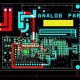# What is Double Tuned Amplifier & Its working?

In this tutorial we are going to learn about what is double Tuned Amplifier & It working?

Double Tuned Amplifier – An Amplifier circuit with a double tuner circuit being at the collector of this circuit is called as double tuner circuit.

Construction of Double Tuned Amplifier –

In double tuned amplifier consist transistor amplifier with two tuned circuit L1C1 has been show as collector load and second is L2C2 as the output. One NPN transistor which collector are connected to L1C1 input , base of transistor are connected to VS voltage through C3 coupling capacitor and emitter are connected to R3 and C4. In single tune amplifier have reduced the bandwidth so that it use overcome this use double tuned amplifier. As per circuit diagram for double tuned amplifier.

Working of Tuned Amplifier –

R1.R2 and RE work as a biasing which is use to provide current and voltage for the transistor operation. When we provide the signal from VS through C3 is coupling capacitor which provides signal at the base of NPN transistor, resonance frequency of first tune circuit L1C1 is equal to signal frequency in that situation tune circuit produce high impedance at input so that large output appear at L1C1 due to this output of L2C2 is inductive couple with first tune circuit so that get best large signal at output. So that at output side will not get bandwidth reduced. Double tuned circuit use to the double tuned voltage amplifier use in intermediate frequency amplifiers in radio and television receiver.

Double Tuned Amplifier Frequency

Frequency response of double tuned amplifier depends upon degree of coupling between two tuned circuits. It means depend on how much are coupling between L1C1 and L2C2. Degree of coupling gives idea of the amount of energy transferred between two tuned circuits. Different type of coupling is in double tuned circuit.

Here below are types of coupling of double tuned amplifier.

1. Tight Coupling – In this coupling tune circuit tightly couple to each other tuned circuit then frequency graph below , in graph three resonance frequency and bandwidth is high in case both are couple are tight couple.
2. Critical Coupling – In this tight coupling both couple generated frequency if we decrease the degree of frequency then both are frequency to each other and merge to each other in that case make one resonance frequency it is called critical coupling.
3. Loose Coupling –  If both tuned circuit coupling are loose in that condition frequency gain height peak become decrease that is called loose coupling

In double tuned maximum use tight cooping because it provide better selectivity and better bandwidth , produce high gain so that we use maximum tight coupling.